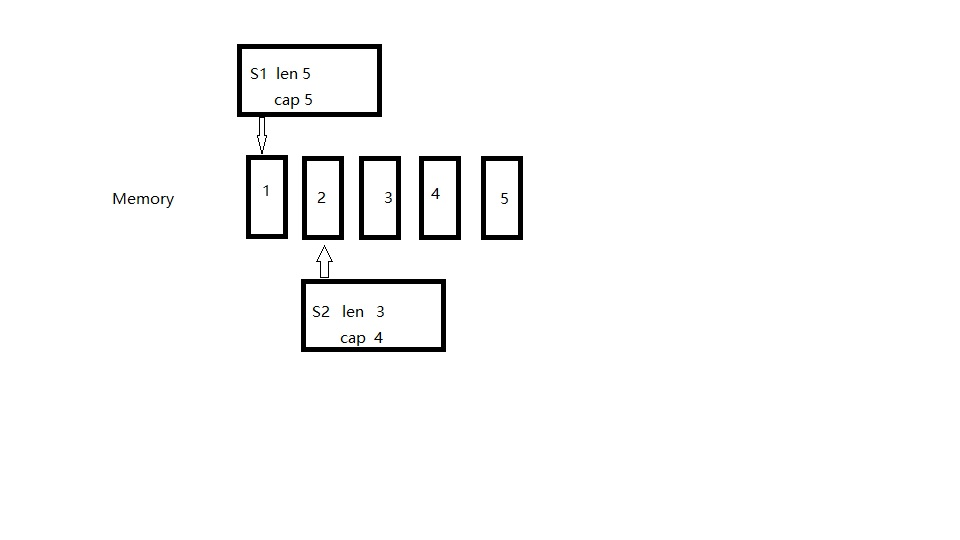func TestAppend(t *testing.T) {
s1 := []int{1, 2, 3, 4, 5}
s2 := s1[0:3]
s2 = append(s2, 30)
fmt.Println(s1, len(s1), cap(s1))
fmt.Println(s2, len(s2), cap(s2))
}

[1 2 3 30 5] 5 5
[2 3 30] 3 4func TestAppend(t *testing.T) {
s1 := []int{1, 2, 3, 4, 5}
s2 := s1[1:3]
s2 = append(s2, 30, 40)
fmt.Println(s1, len(s1), cap(s1))
fmt.Println(s2, len(s2), cap(s2))
}

[1 2 3 30 40] 5 5
[2 3 30 40] 4 4

func TestAppend(t *testing.T) {
s1 := []int{1, 2, 3, 4, 5}
s2 := s1[1:3]
s2 = append(s2, 30, 40, 50)
fmt.Println(s1, len(s1), cap(s1))
fmt.Println(s2, len(s2), cap(s2))
}

[1 2 3 4 5] 5 5
[2 3 30 40 50] 5 8

func TestAppend2(t *testing.T) {
var tmp []int
s2 := []int{1, 2, 3, 4}
fmt.Print(cap(s2), ",")
tmp = make([]int, 2)
s2 = append(s2, tmp...)
fmt.Print(cap(s2), ",")
tmp = make([]int, 4)
s2 = append(s2, tmp...)
fmt.Print(cap(s2), ",")
tmp = make([]int, 6)
s2 = append(s2, tmp...)
fmt.Print(cap(s2), ",")
tmp = make([]int, 7)
s2 = append(s2, tmp...)
fmt.Println(cap(s2), ",")
}

4,8,16,16,32

func TestAppend(t *testing.T) {
s1 := []int{1, 2, 3, 4, 5}
s2 := s1[1:3]
s2 = append(s2, 30)
fmt.Println(s1, len(s1), cap(s1))
fmt.Println(s2, len(s2), cap(s2))
s2 = append(s2, 40)
fmt.Println(s1, len(s1), cap(s1))
fmt.Println(s2, len(s2), cap(s2))
s2 = append(s2, 50)
fmt.Println(s1, len(s1), cap(s1))
fmt.Println(s2, len(s2), cap(s2))
}

[1 2 3 30 5] 5 5
[2 3 30] 3 4
[1 2 3 30 40] 5 5
[2 3 30 40] 4 4
[1 2 3 30 40] 5 5
[2 3 30 40 50] 5 8